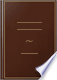Søk Bilder Maps Play YouTube Nyheter Gmail Disk Mer »
Logg på
 Bøker Bok 11–20 av 165 på ANY two angles of a triangle are together less than two right angles.ANY two angles of a triangle are together less than two right angles.The Elements of Euclid - Side 20
av Euclid - 1838 - 416 sider
Uten tilgangsbegrensning - Om denne boken## Elements of Geometry: Containing the First Six Books of Euclid, with a ...

John Playfair - 1819 - 333 sider
...&c. Q, ED PROP. XVII. THEOR. Jlny too angles of a triangle are together less than two right angle's. Let ABC be any triangle ; any two of its angles together are less than two right angles. Produce BC'to D ; and because ACD is the exterior angle of the triangle ABC, ACD is greater (16. 1.) than the...
Uten tilgangsbegrensning - Om denne boken## Elements of Geometry: Containing the First Six Books of Euclid: With a ...

John Playfair - 1819 - 317 sider
...BCG, that is (15. 1.), the angle ACD, is greater than the angle ABC. Therefore, if one side, &c. Q, ED PROP. XVII. THEOR. - Any two angles of a triangle are together less than' two right angle*. Let A BC be any triangle ; any two of its angles together are less than two right angles. Produce...
Uten tilgangsbegrensning - Om denne boken## The Youth's instructer [sic] and guardian

1854
...proved hereafter, it is here inserted in order to facilitate the theory of parallel lines. Cor. 2. Any two angles of a triangle are together less than two right angles. For either is less than the supplement of the other. Cor. 3. If any angle of a triangle is right or...
Uten tilgangsbegrensning - Om denne boken## Elements of Geometry: Containing the First Six Books of Euclid, with a ...

Euclid, John Playfair - 1826 - 320 sider
...if one Bide, <SeQED 4 PROP. XVII. t HEOR. Any two angles of a triangle are together less than twfl right angles. Let ABC be any triangle; any two of...its angles together are less than two right angles. Produee BC to D ; and beeause ACD is the exterior angle of the triangle ABC, ACD, is greater (16. 1.)...
Uten tilgangsbegrensning - Om denne boken## Elements of arithmetic, algebra and geometry

George Lees - 1826 - 207 sider
...that the angle BCG, that is, ACD% is e 5. 1. greater than ABC. Therefore, if one side, &c. QED Cor. Any two angles of a triangle, are together less than two right angles. For, since by the proposition, the exterior angle ACD is greater than the interior and opposite angle...
Uten tilgangsbegrensning - Om denne boken## Geometry, Plane, Solid, and Spherical, in Six Books: To which is Added, in ...

Pierce Morton - 1830 - 272 sider
...the one equal to the three sides of the other, each to each, are equul in all respects . . 7 (•7) Any two angles of a triangle are together less than two right angles 7 (A} A triangle cannot have more than one right angle, or more than one obtuse angle . . nor. 7 (i)...
Uten tilgangsbegrensning - Om denne boken## On the study and difficulties of mathematics [by A. De Morgan].

Augustus De Morgan - 1831
...than two right angles, is not an assumption but a consequence of his proposition, which shews that any two angles of a triangle are together less than two right angles, and which is established before any mention is made of parallels. It has been found by the experience...
Uten tilgangsbegrensning - Om denne boken## The Elements of geometry [Euclid book 1-3] in general terms, with ..., Del 3

Euclides - 1833
...line. For then an external angle would be = to an internal and opposite, which is absurd. PROP. 17, THEOR. Any two angles of a triangle are together less than two right angles. Produce any side ; then the external angle is greater than either of the internal remote angles ; but...
Uten tilgangsbegrensning - Om denne boken## Geometry Without Axioms; Or the First Book of Euclid's Elements. With ...

Thomas Perronet Thompson - 1833 - 150 sider
...And by parity of reasoning, the like may be proved in every other triangle. Wherefore, universally, any two angles of a triangle are together less than two right angles. Which was to be demonstrated. COH. 1. A triangle cannot have more than one right angle; nor more than...
Uten tilgangsbegrensning - Om denne boken## The Elements of Euclid; viz. the first six books, together with the eleventh ...

Euclides - 1834
...side, &e. OED PROPOSITION XVII. THEOR. — Any two angles of a triangle are together lest than Iwo right angles. Let ABC be any triangle : any two of its angles together shall be less than two right angles. Produce BC to D : and because ACD is the exterior angle of the...
Uten tilgangsbegrensning - Om denne boken